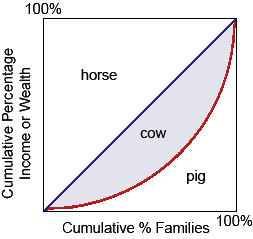Marginal Resource Costs problem

Can someone please help me in finding out the accurate answer from the following question. The synonymous words of marginal factor costs or marginal resource costs signify to the: (i) Cost incurred in generating an extra unit of capital. (ii) Cost to the resource owner of securing employment for resource. (iii) Additional cost included in hiring an extra unit of a resource. (iv) Modification in total cost related with the production of one more extra unit of output.

Related Questions in Microeconomics

• Q :Difference among change in

The difference between change in supply and change in quantity supplied is as follows: (1) The change in quantity supplied is caused just by the change in the price of good, whereas a change in supply takes place whenever the ceteris paribus suppositi

• Q :Why demand curve face monopolistically

Why is demand curve facing a monopolistically competitive firm probable to be very elastic?

• Q :Asset demand Select the right answer of

Select the right answer of the question. The asset demand for money: A) is unrelated to both the interest rate and the level of GDP. B) varies inversely with the rate of interest. C) varies inversely with the level of real GDP. D) varies directly with the level of nom

• Q :Higher interest rate in current

For current consumption growing preferences over future consumption would be evidenced from a: (w) higher interest rate. (x) more quick rate of investment. (y) larger government budget surplus. (z) surplus into the balance of trade.

• Q :Demonstrates the Lorenz Curve This

This given figure demonstrates as: (w) Lorenz curve. (x) familial income distribution graph. (y) Gini curve. (z) Blanc income standard curve.Q :Analytic time in the market period In

In the market period: (w) price is constant. (x) output is constant. (y) supply is horizontal. (z) supply is completely elastic.

Please guys help to solve this problem of Economics with some explan

• Q :Calls of negative income tax Negative

Negative income tax proposals call for: (w) paying \$1 in taxes on every dollar of transfer payments. (x) reducing welfare benefits by levying higher income taxes. (y) instituting consistent work incentives for welfare recipients. (z) establishing a ne

• Q :Marginal factor cost The Siberian

The Siberian Software vends custom programs to big multinationals. Its programs are coded in the remote region. In equilibrium, the Siberian faces a marginal factor cost for the programmers of roughly: (1) \$21 per hour. (2) \$24 per hour. (3) \$12 per hour. (4) \$18 per

• Q :Total revenue when a price increases

For hamburgers the demand is relatively elastic. When the price of hamburgers increases, in that case: (i) the quantity demanded will go up. (ii) its demand will increase. (iii) total revenue will increase. (iv) total revenue will reduce.

• Q :Minimum Wage Laws and Monopsony Power

The Minimum wage laws potentially raise both employment and wages if firms: (i) Have monopsony power in the labor market and don’t wage discriminate. (ii) Practice outsourcing across the international borders as labor costs abroad are lower. (iii) Are pure compe